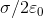# Problem: Please answer True or False for the following questions:1.) Gauss' law is useful for calculating the electric field when the charge distribution is highly symmetrical.2.) At electrostatic equilibrium, the electric field is zero everywhere inside a conductor, and any charge can only be distributed on the surface of the conductor.3.) Electric field just outside a charged conductor is perpendicular to the surface and has a magnitude Please provide an explanation for your responses.

###### FREE Expert Solution

1.)

Flux through a surface:

$\overline{){\mathbf{\varphi }}{\mathbf{=}}\frac{{\mathbf{q}}_{\mathbf{e}\mathbf{n}\mathbf{c}\mathbf{l}\mathbf{o}\mathbf{s}\mathbf{e}\mathbf{d}}}{{\mathbf{\epsilon }}_{\mathbf{0}}}}$

86% (460 ratings)###### Problem Details

1.) Gauss' law is useful for calculating the electric field when the charge distribution is highly symmetrical.

2.) At electrostatic equilibrium, the electric field is zero everywhere inside a conductor, and any charge can only be distributed on the surface of the conductor.

3.) Electric field just outside a charged conductor is perpendicular to the surface and has a magnitude